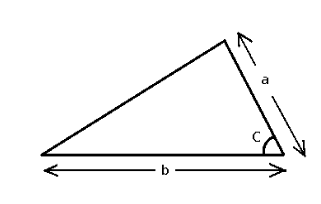# SIDE ANGLE SIDE FORMULA

When 2 sides and an angle of the triangle are already provided, then, by using the sine trigonometric function, side angle side formula is used to calculate the area of the triangle. In short, we call this formula as “SAS” when the two sides and the angle between them is given.

There are mainly three steps in calculating the required angles of the triangle:

• Step 1. We first find the unknown side using the Law of Cosine.
• Step 2. To find the smallest of the two angles, we use the Law of Sines
• Step 3. Then add the three angles to 180 degrees and find the third angle.The Side angle side formula is given as

$\large Area=\frac{ab\;Sin\,C}{2}$

### Solved example

Question: What will be the area of a triangle whose sides are of length 6 cm and 10 cm and its included angle is 45 degrees ?

Solution:

Using the formula:

$$\begin{array}{l}Area=\frac{ab\;Sin\,C}{2}\end{array}$$
$$\begin{array}{l}Area=\frac{6\times 10\times sin 45^{\circ}}{2}\end{array}$$
$$\begin{array}{l}=\frac{60\times 0.70}{2}\end{array}$$
$$\begin{array}{l}= 21\;cm^{2}\end{array}$$